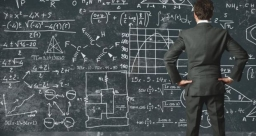# Numbers 24281

Of the four numbers, the second is five larger than the first, the third two smaller than the first, and the fourth is twice larger than the first. Find these numbers if their sum is 38.

a =  7
b =  12
c =  5
d =  14

### Step-by-step explanation:

b=5+a
c =a-2
d=2a
a+b+c+d=38

b=5+a
c =a-2
d=2·a
a+b+c+d=38

a-b = -5
a-c = 2
2a-d = 0
a+b+c+d = 38

Pivot: Row 1 ↔ Row 3
2a-d = 0
a-c = 2
a-b = -5
a+b+c+d = 38

Row 2 - 1/2 · Row 1 → Row 2
2a-d = 0
-c+0.5d = 2
a-b = -5
a+b+c+d = 38

Row 3 - 1/2 · Row 1 → Row 3
2a-d = 0
-c+0.5d = 2
-b+0.5d = -5
a+b+c+d = 38

Row 4 - 1/2 · Row 1 → Row 4
2a-d = 0
-c+0.5d = 2
-b+0.5d = -5
b+c+1.5d = 38

Pivot: Row 2 ↔ Row 3
2a-d = 0
-b+0.5d = -5
-c+0.5d = 2
b+c+1.5d = 38

Row 4 + Row 2 → Row 4
2a-d = 0
-b+0.5d = -5
-c+0.5d = 2
c+2d = 33

Row 4 + Row 3 → Row 4
2a-d = 0
-b+0.5d = -5
-c+0.5d = 2
2.5d = 35

d = 35/2.5 = 14
c = 2-0.5d/-1 = 2-0.5 · 14/-1 = 5
b = -5-0.5d/-1 = -5-0.5 · 14/-1 = 12
a = 0+d/2 = 0+14/2 = 7

a = 7
b = 12
c = 5
d = 14

Our linear equations calculator calculates it.Did you find an error or inaccuracy? Feel free to write us. Thank you!

Tips for related online calculators
Do you have a system of equations and looking for calculator system of linear equations?